Скачать презентацию 3 Working With Financial Statements Mc Graw-Hill Irwin Copyright

265c4939c639cc161a7f647aeee4dd3d.ppt

• Количество слайдов: 67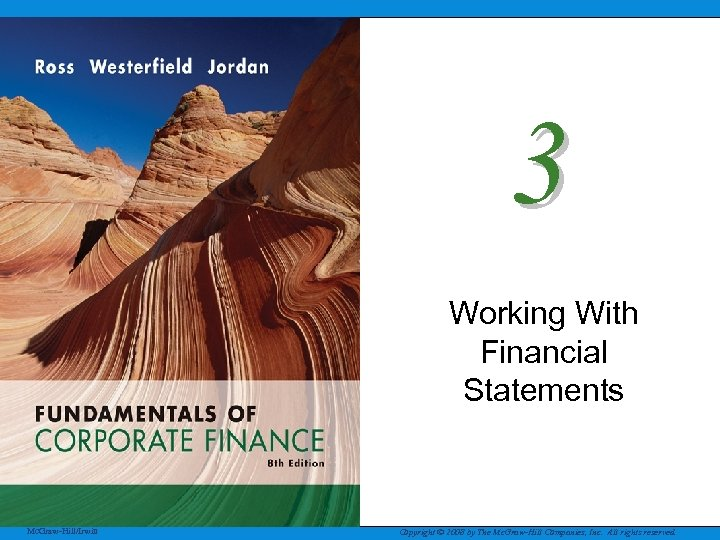3 Working With Financial Statements Mc. Graw-Hill/Irwin Copyright © 2008 by The Mc. Graw-Hill Companies, Inc. All rights reserved.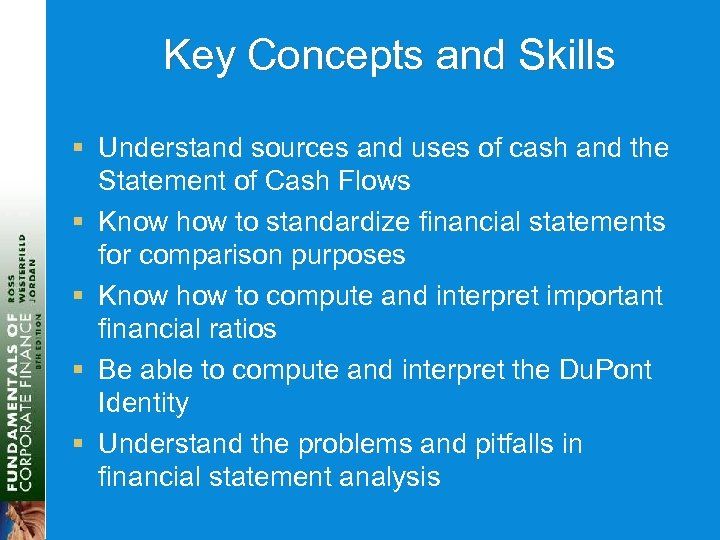Key Concepts and Skills § Understand sources and uses of cash and the Statement of Cash Flows § Know how to standardize financial statements for comparison purposes § Know how to compute and interpret important financial ratios § Be able to compute and interpret the Du. Pont Identity § Understand the problems and pitfalls in financial statement analysis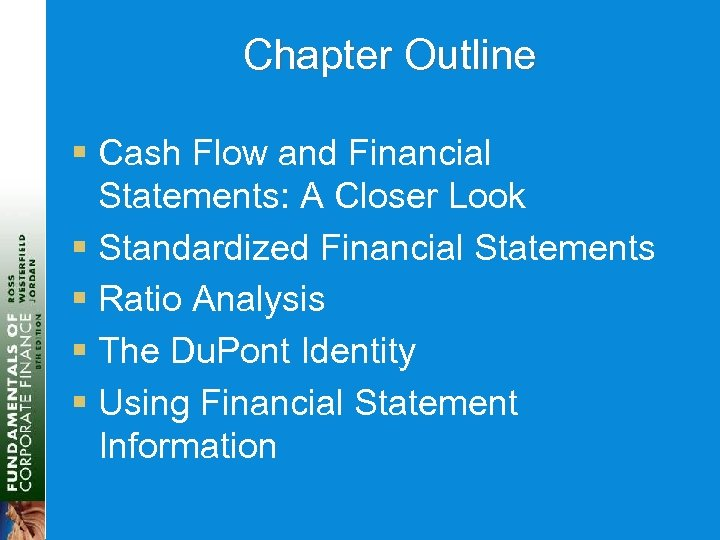Chapter Outline § Cash Flow and Financial Statements: A Closer Look § Standardized Financial Statements § Ratio Analysis § The Du. Pont Identity § Using Financial Statement Information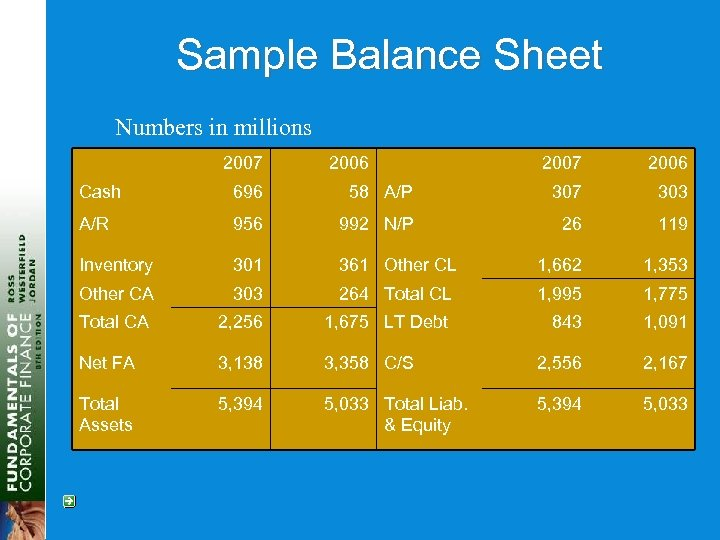Sample Balance Sheet Numbers in millions 2007 2006 Cash 696 58 A/P 307 303 A/R 956 992 N/P 26 119 Inventory 301 361 Other CL 1, 662 1, 353 Other CA 303 264 Total CL 1, 995 1, 775 Total CA 2, 256 1, 675 LT Debt 843 1, 091 Net FA 3, 138 3, 358 C/S 2, 556 2, 167 Total Assets 5, 394 5, 033 Total Liab. & Equity 5, 394 5, 033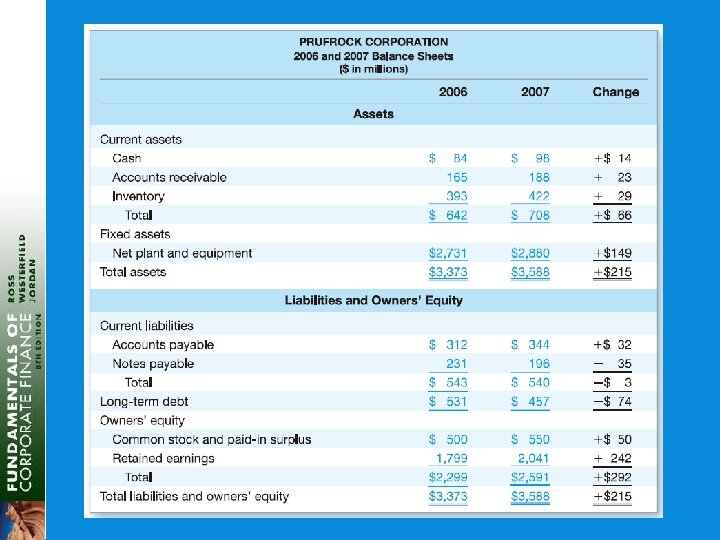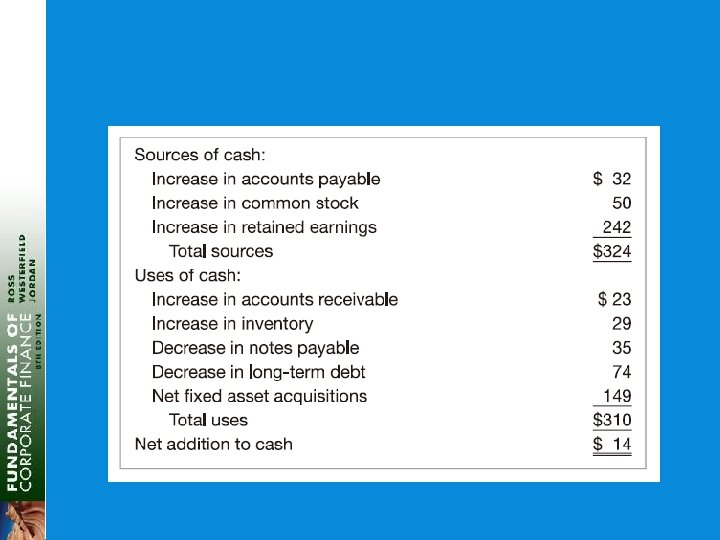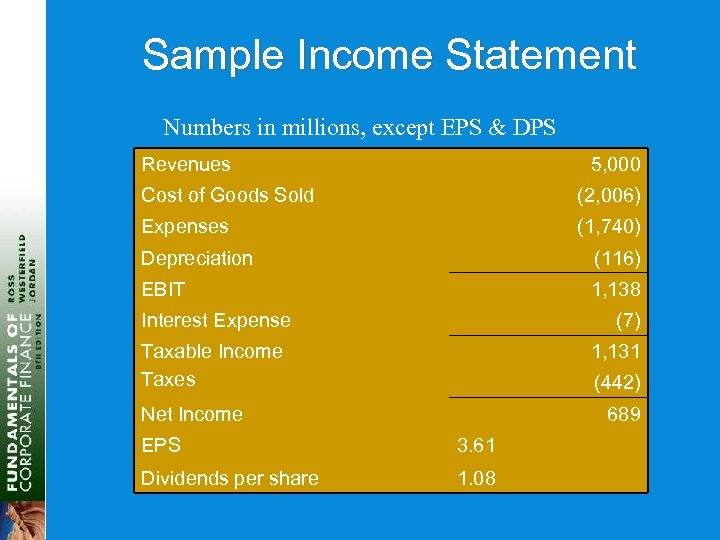Sample Income Statement Numbers in millions, except EPS & DPS Revenues 5, 000 Cost of Goods Sold (2, 006) Expenses (1, 740) Depreciation (116) EBIT 1, 138 Interest Expense (7) Taxable Income Taxes 1, 131 (442) Net Income 689 EPS 3. 61 Dividends per share 1. 08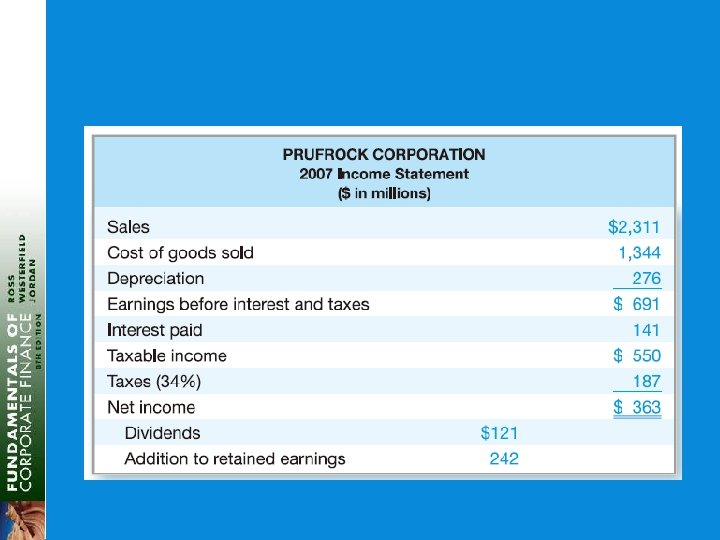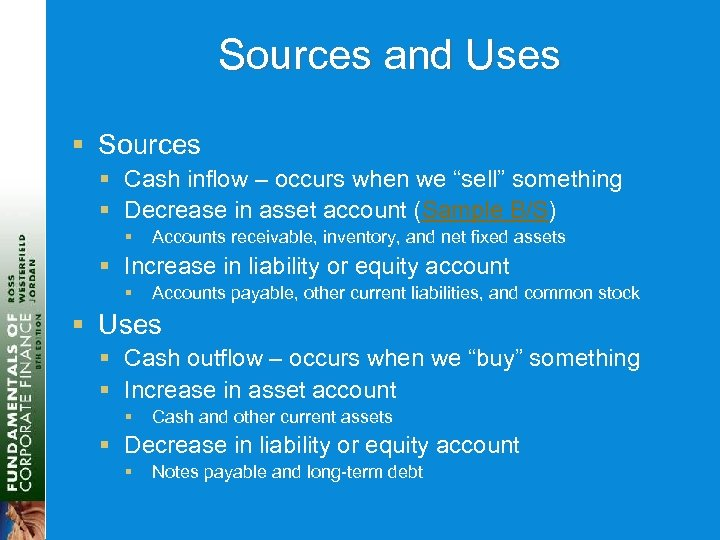Sources and Uses § Sources § Cash inflow – occurs when we “sell” something § Decrease in asset account (Sample B/S) § Accounts receivable, inventory, and net fixed assets § Increase in liability or equity account § Accounts payable, other current liabilities, and common stock § Uses § Cash outflow – occurs when we “buy” something § Increase in asset account § Cash and other current assets § Decrease in liability or equity account § Notes payable and long-term debt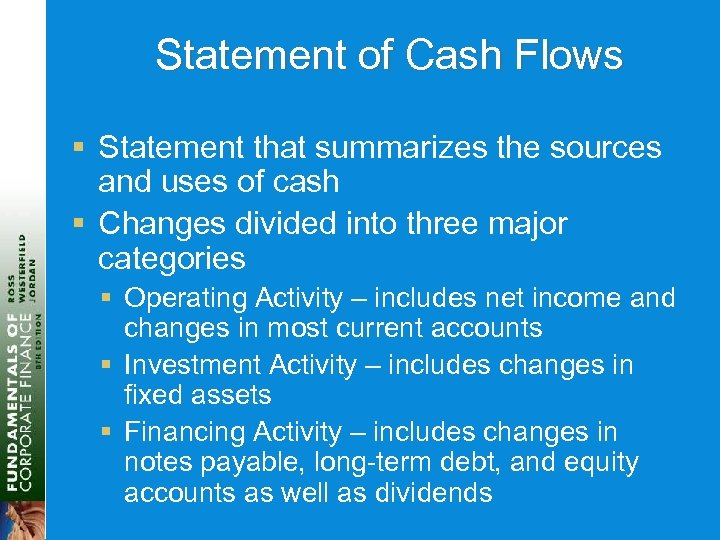Statement of Cash Flows § Statement that summarizes the sources and uses of cash § Changes divided into three major categories § Operating Activity – includes net income and changes in most current accounts § Investment Activity – includes changes in fixed assets § Financing Activity – includes changes in notes payable, long-term debt, and equity accounts as well as dividends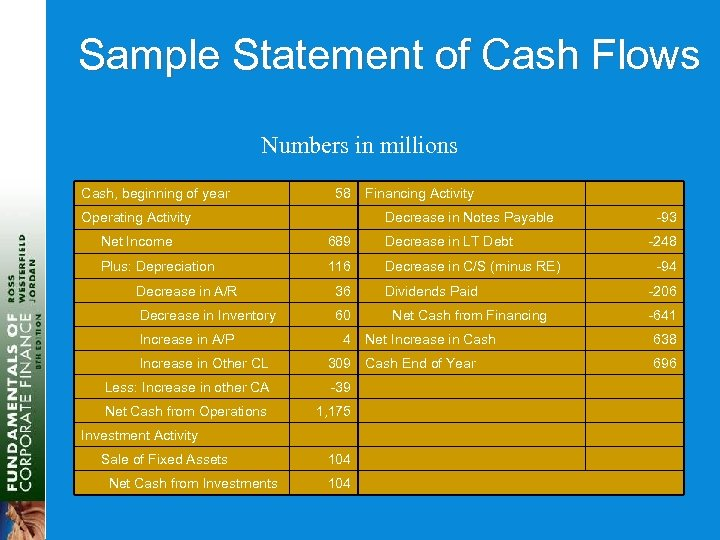Sample Statement of Cash Flows Numbers in millions Cash, beginning of year 58 Operating Activity Financing Activity Decrease in Notes Payable Net Income 689 Decrease in LT Debt Plus: Depreciation 116 Decrease in C/S (minus RE) Decrease in A/R 36 Decrease in Inventory 60 Increase in A/P 4 Increase in Other CL 309 Less: Increase in other CA -39 Net Cash from Operations 1, 175 Investment Activity Sale of Fixed Assets Net Cash from Investments 104 Dividends Paid Net Cash from Financing -93 -248 -94 -206 -641 Net Increase in Cash 638 Cash End of Year 696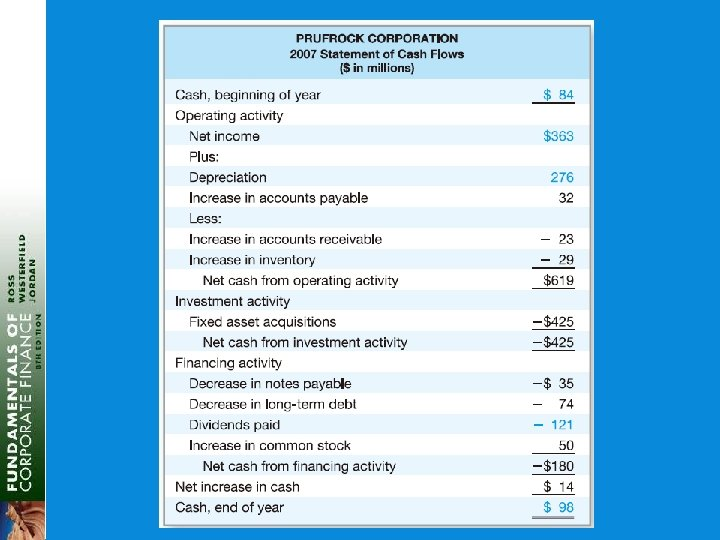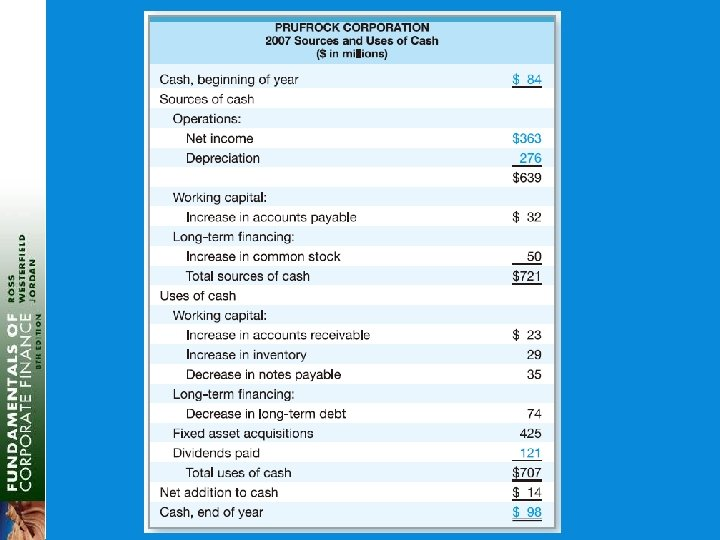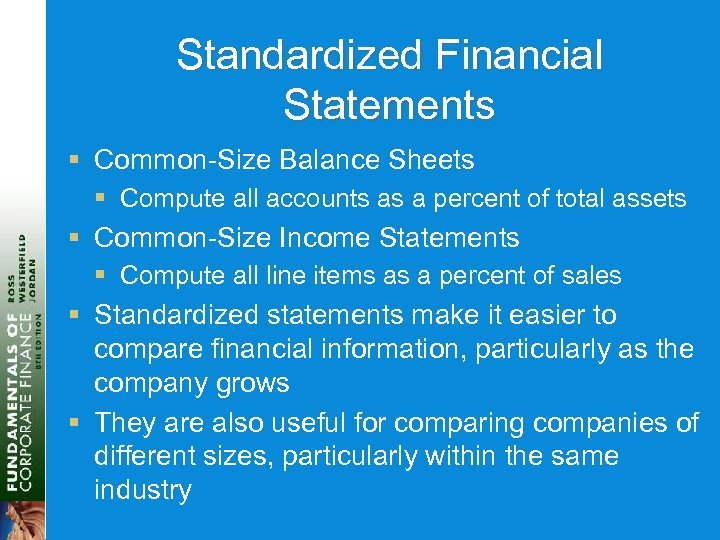Standardized Financial Statements § Common-Size Balance Sheets § Compute all accounts as a percent of total assets § Common-Size Income Statements § Compute all line items as a percent of sales § Standardized statements make it easier to compare financial information, particularly as the company grows § They are also useful for comparing companies of different sizes, particularly within the same industry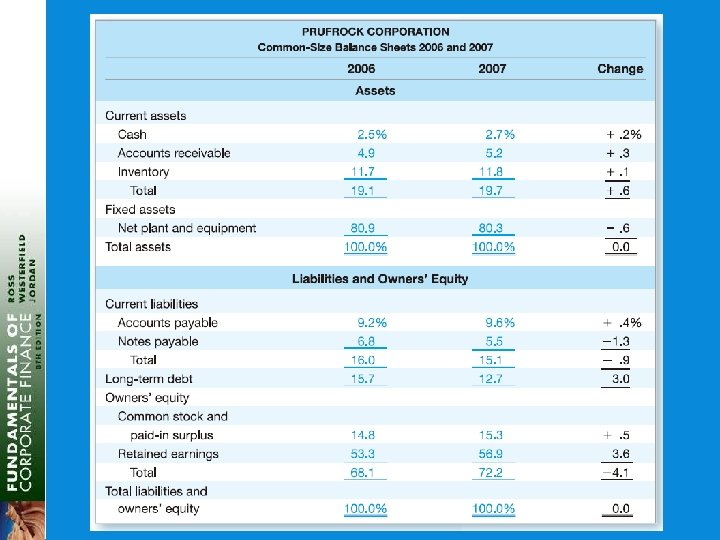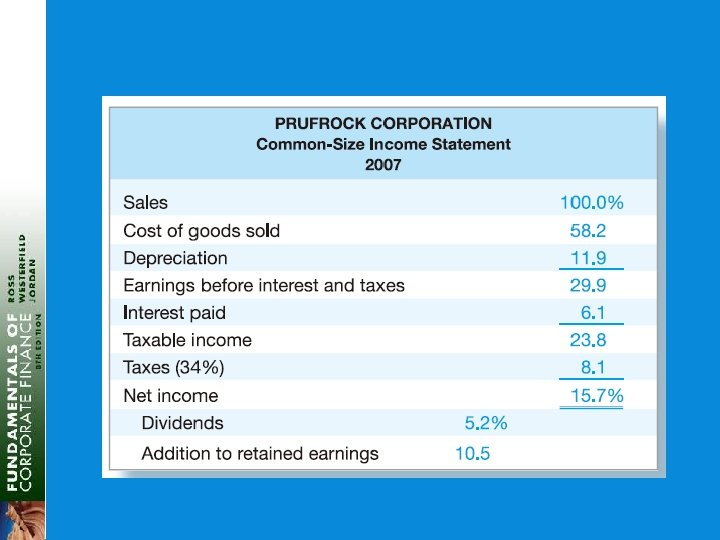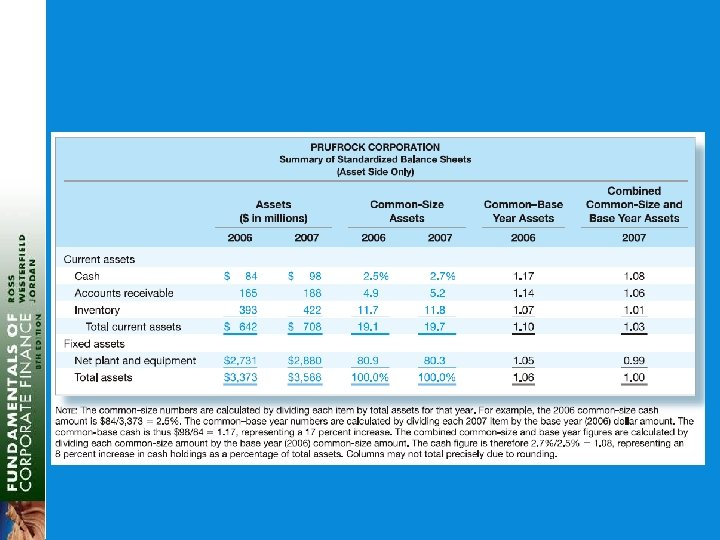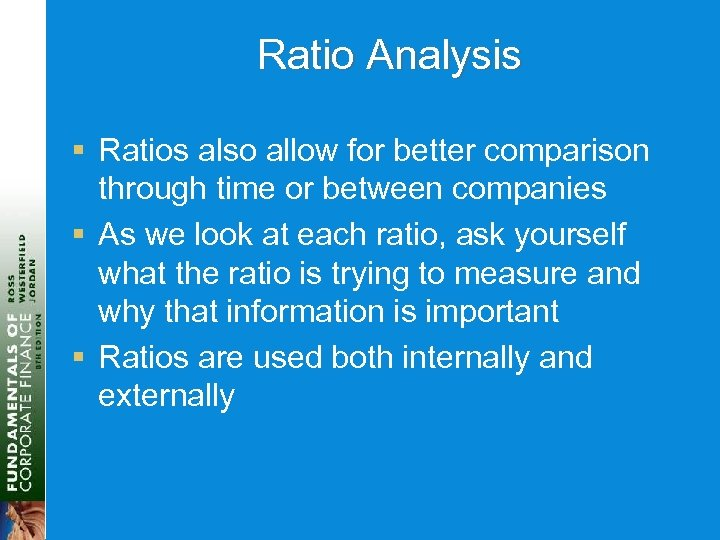Ratio Analysis § Ratios also allow for better comparison through time or between companies § As we look at each ratio, ask yourself what the ratio is trying to measure and why that information is important § Ratios are used both internally and externally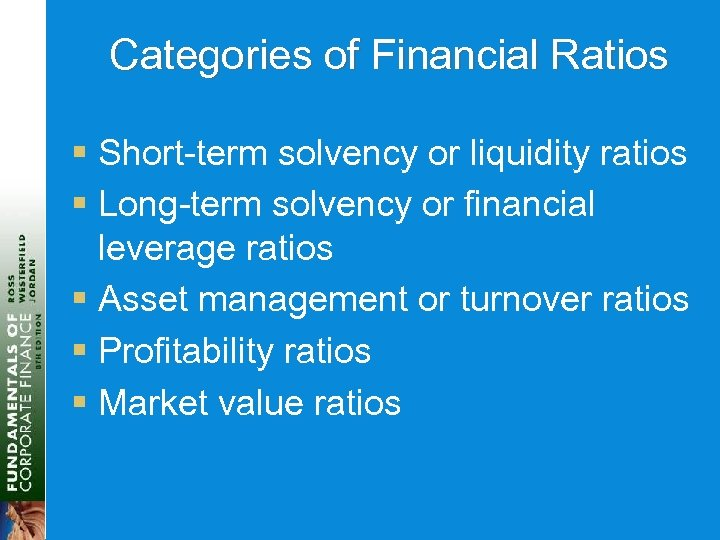Categories of Financial Ratios § Short-term solvency or liquidity ratios § Long-term solvency or financial leverage ratios § Asset management or turnover ratios § Profitability ratios § Market value ratios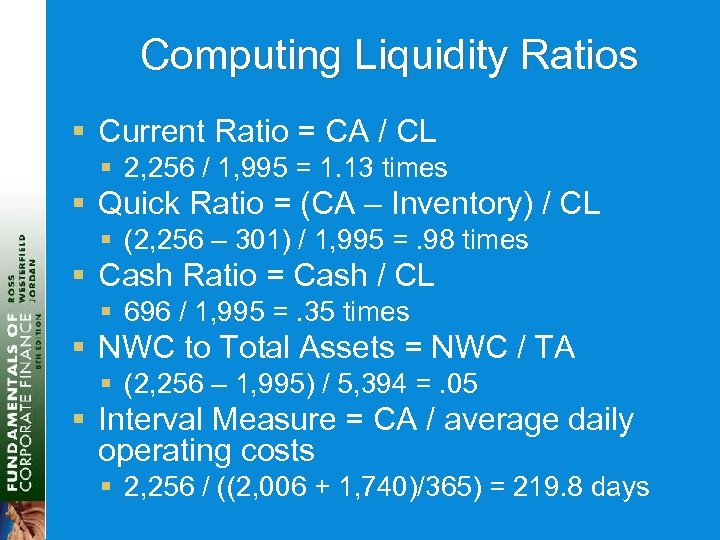Computing Liquidity Ratios § Current Ratio = CA / CL § 2, 256 / 1, 995 = 1. 13 times § Quick Ratio = (CA – Inventory) / CL § (2, 256 – 301) / 1, 995 =. 98 times § Cash Ratio = Cash / CL § 696 / 1, 995 =. 35 times § NWC to Total Assets = NWC / TA § (2, 256 – 1, 995) / 5, 394 =. 05 § Interval Measure = CA / average daily operating costs § 2, 256 / ((2, 006 + 1, 740)/365) = 219. 8 days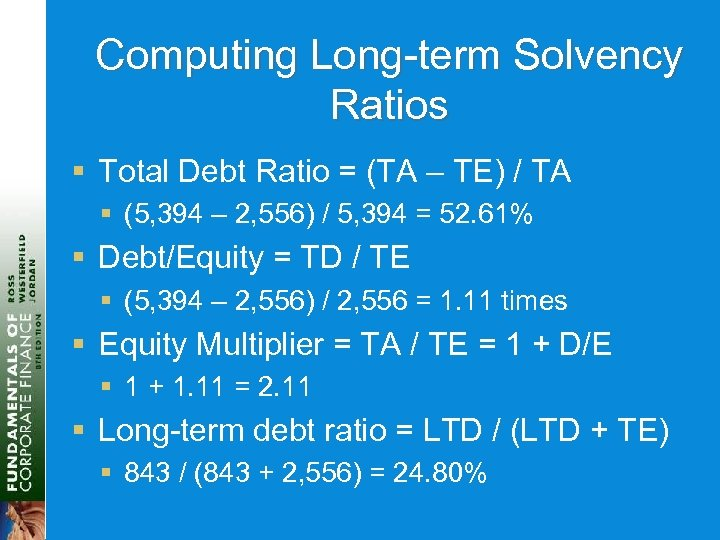Computing Long-term Solvency Ratios § Total Debt Ratio = (TA – TE) / TA § (5, 394 – 2, 556) / 5, 394 = 52. 61% § Debt/Equity = TD / TE § (5, 394 – 2, 556) / 2, 556 = 1. 11 times § Equity Multiplier = TA / TE = 1 + D/E § 1 + 1. 11 = 2. 11 § Long-term debt ratio = LTD / (LTD + TE) § 843 / (843 + 2, 556) = 24. 80%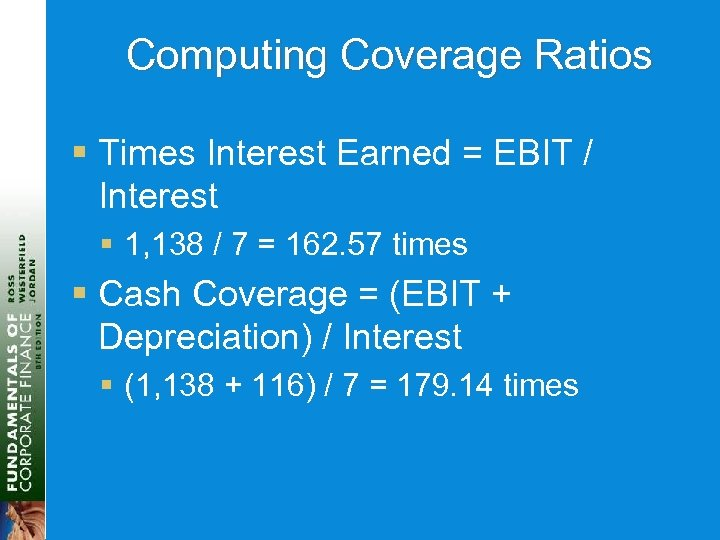Computing Coverage Ratios § Times Interest Earned = EBIT / Interest § 1, 138 / 7 = 162. 57 times § Cash Coverage = (EBIT + Depreciation) / Interest § (1, 138 + 116) / 7 = 179. 14 times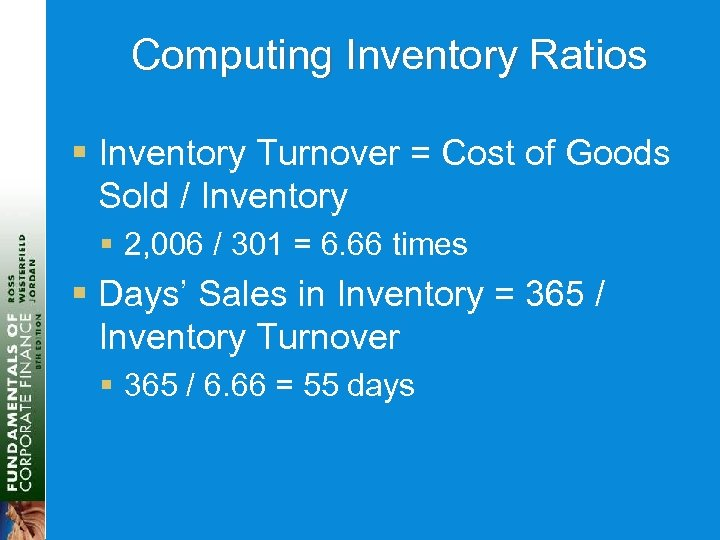Computing Inventory Ratios § Inventory Turnover = Cost of Goods Sold / Inventory § 2, 006 / 301 = 6. 66 times § Days’ Sales in Inventory = 365 / Inventory Turnover § 365 / 6. 66 = 55 days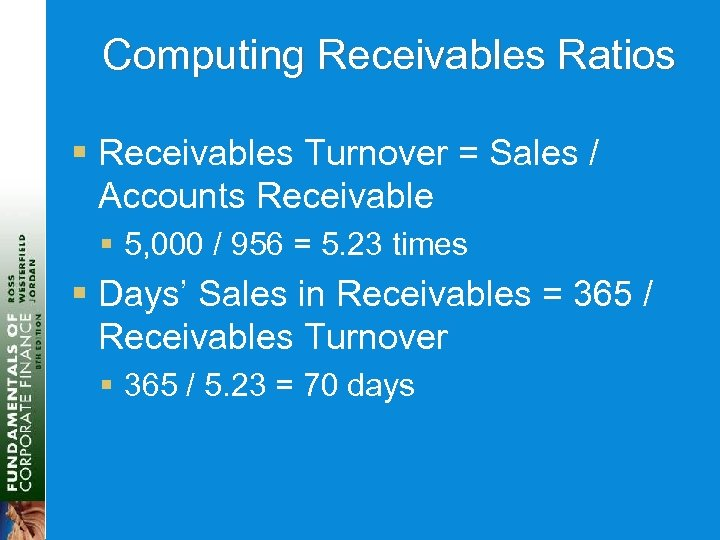Computing Receivables Ratios § Receivables Turnover = Sales / Accounts Receivable § 5, 000 / 956 = 5. 23 times § Days’ Sales in Receivables = 365 / Receivables Turnover § 365 / 5. 23 = 70 days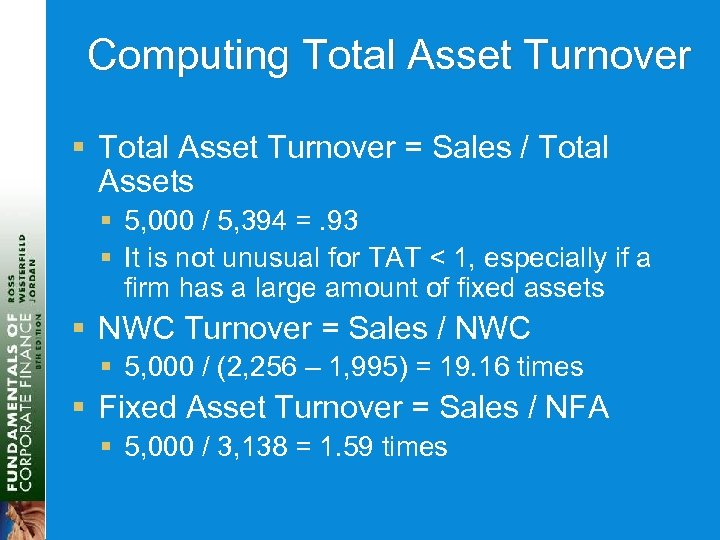Computing Total Asset Turnover § Total Asset Turnover = Sales / Total Assets § 5, 000 / 5, 394 =. 93 § It is not unusual for TAT < 1, especially if a firm has a large amount of fixed assets § NWC Turnover = Sales / NWC § 5, 000 / (2, 256 – 1, 995) = 19. 16 times § Fixed Asset Turnover = Sales / NFA § 5, 000 / 3, 138 = 1. 59 times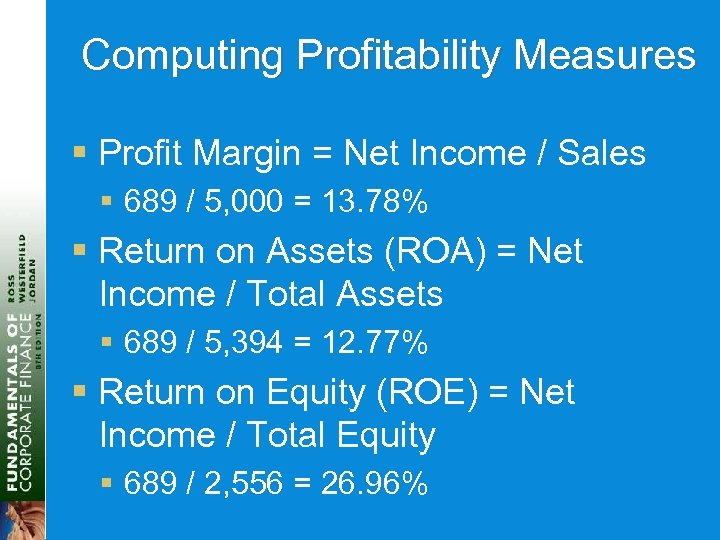Computing Profitability Measures § Profit Margin = Net Income / Sales § 689 / 5, 000 = 13. 78% § Return on Assets (ROA) = Net Income / Total Assets § 689 / 5, 394 = 12. 77% § Return on Equity (ROE) = Net Income / Total Equity § 689 / 2, 556 = 26. 96%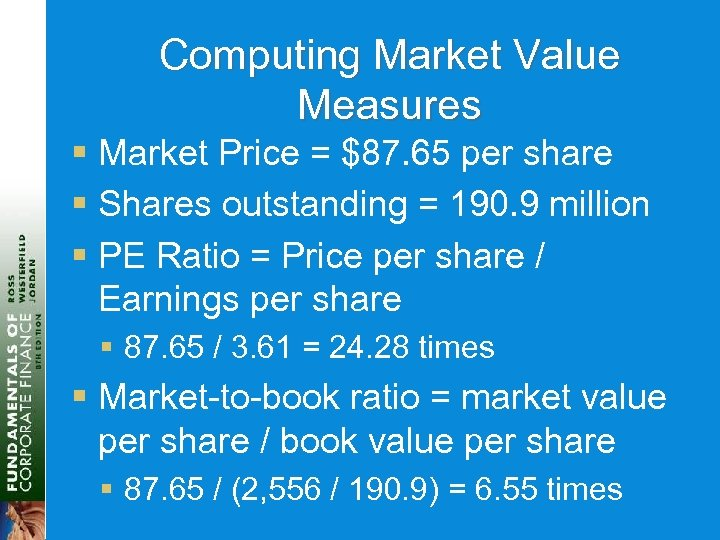Computing Market Value Measures § Market Price = \$87. 65 per share § Shares outstanding = 190. 9 million § PE Ratio = Price per share / Earnings per share § 87. 65 / 3. 61 = 24. 28 times § Market-to-book ratio = market value per share / book value per share § 87. 65 / (2, 556 / 190. 9) = 6. 55 times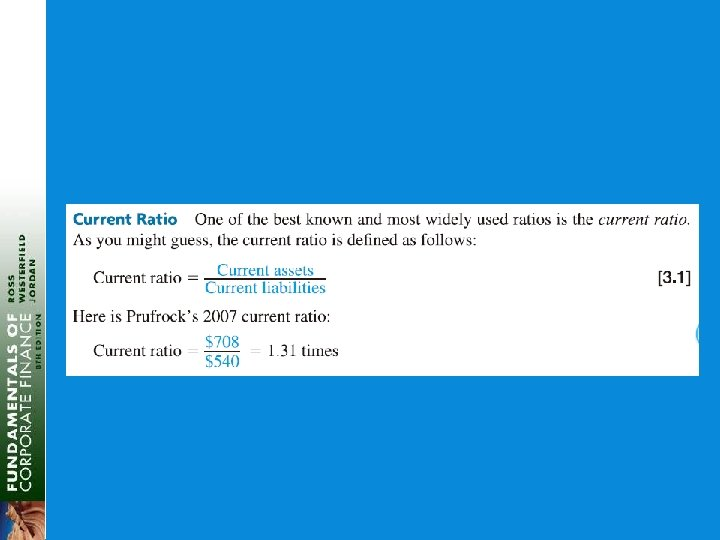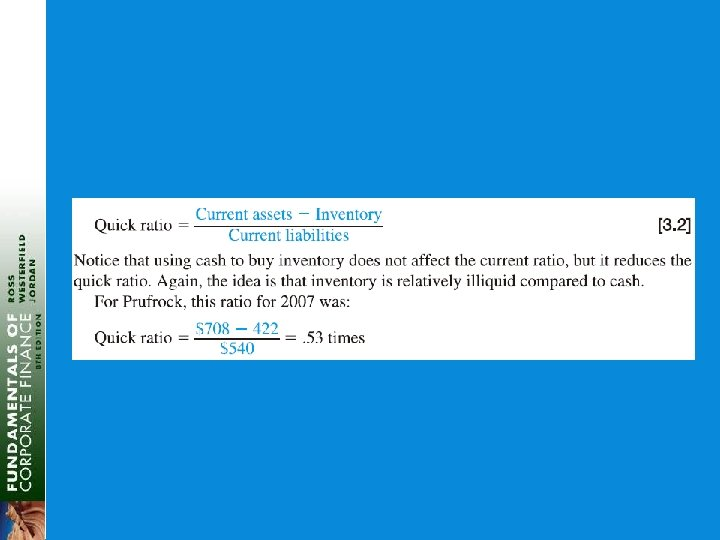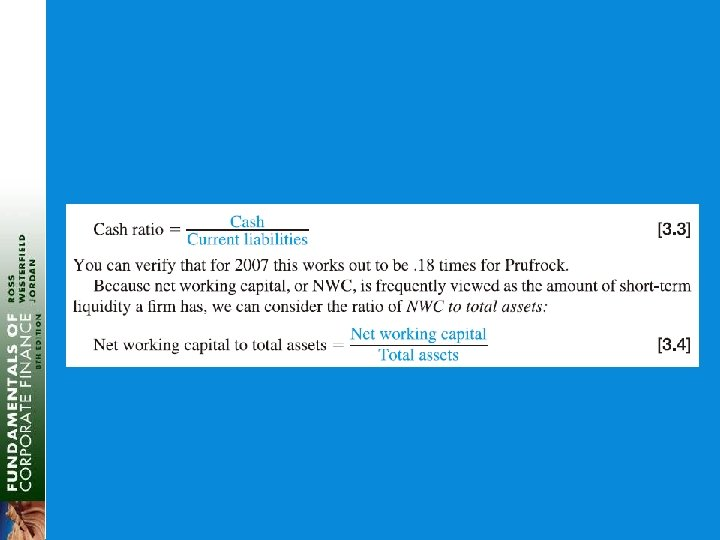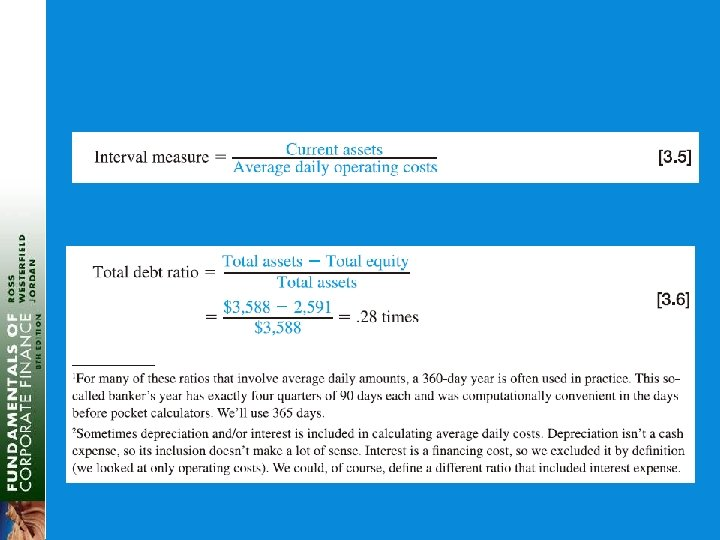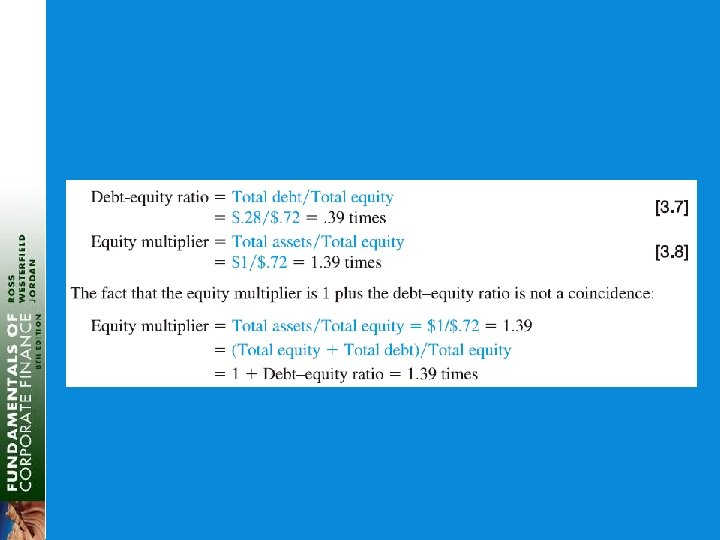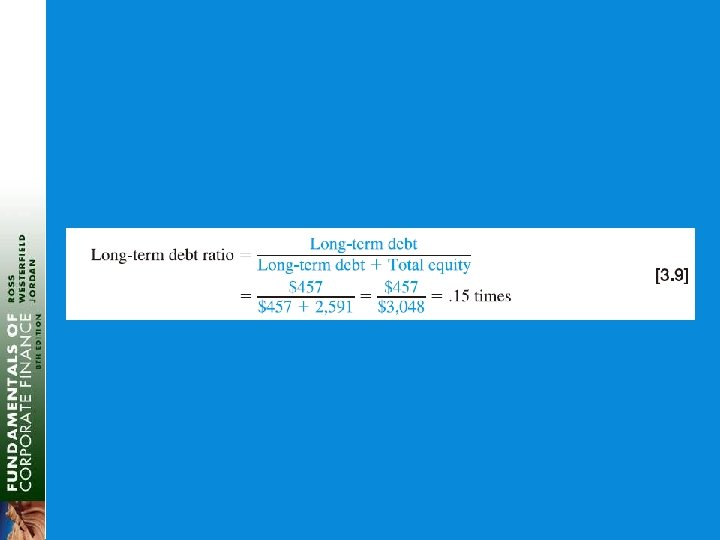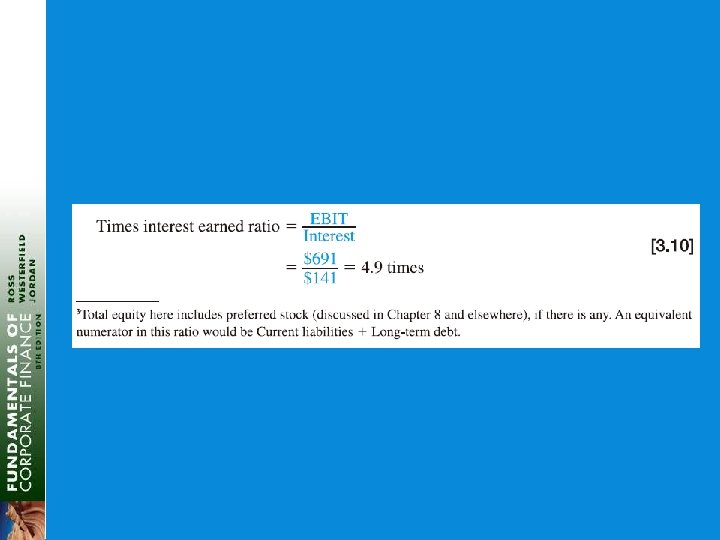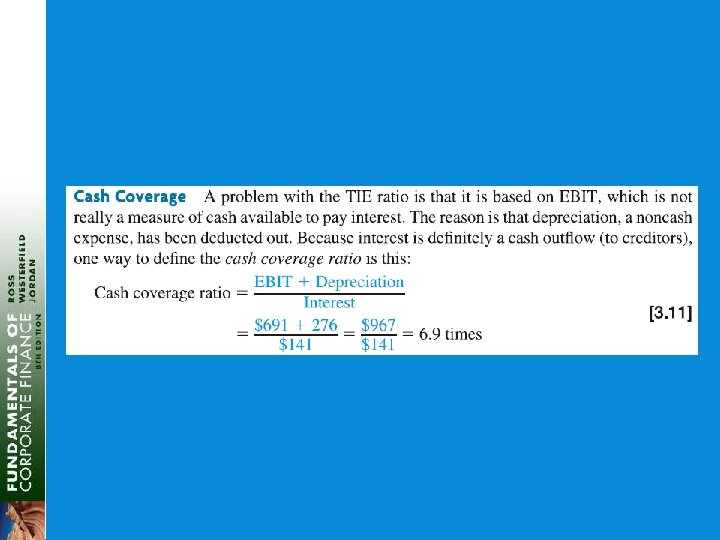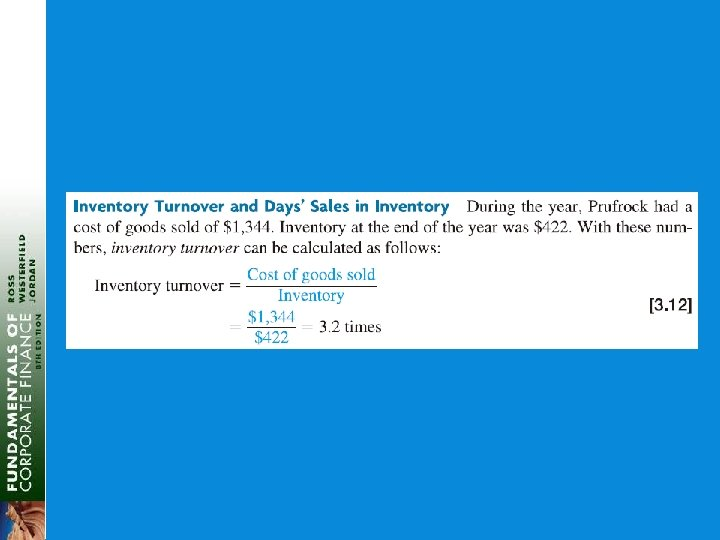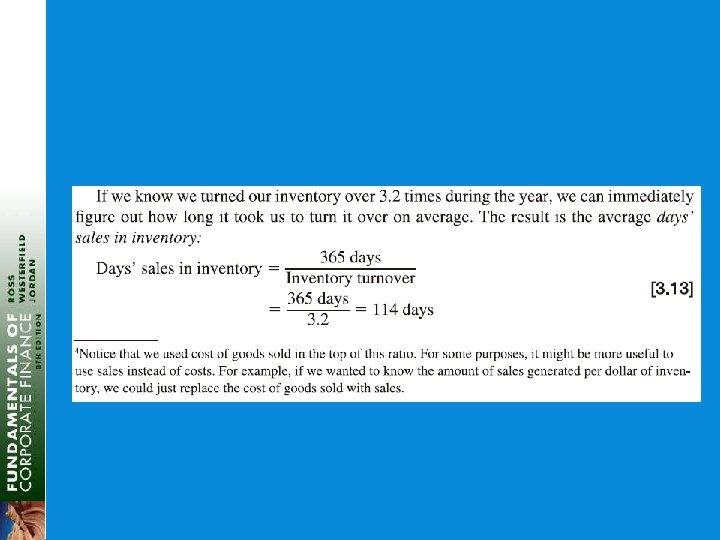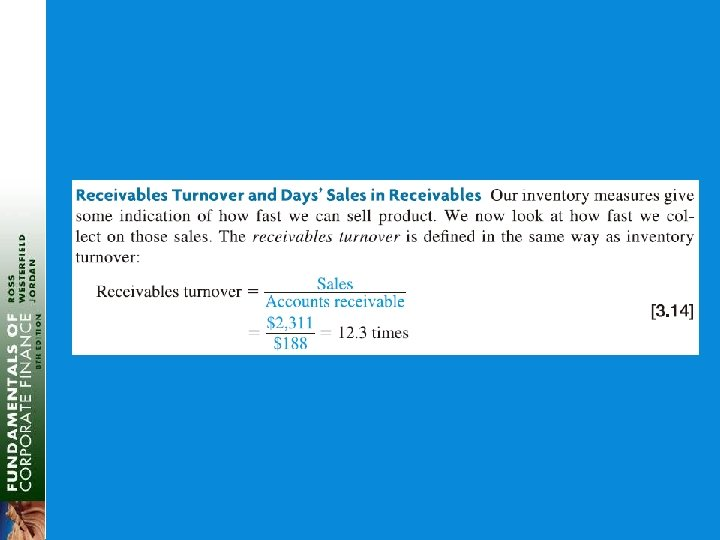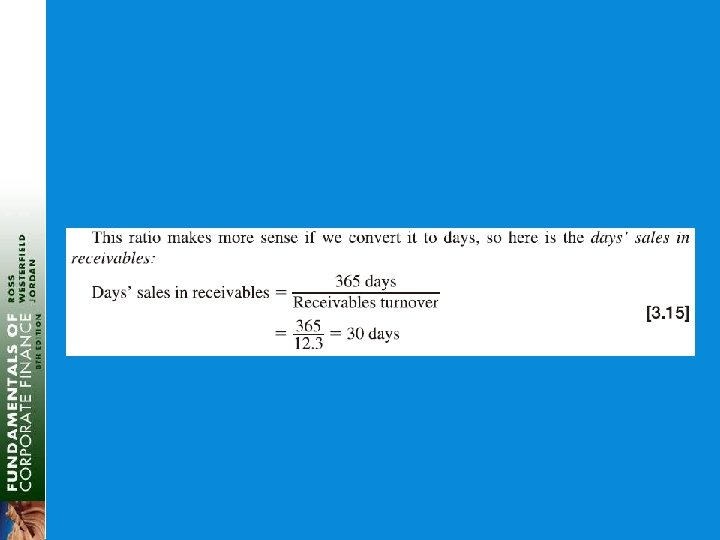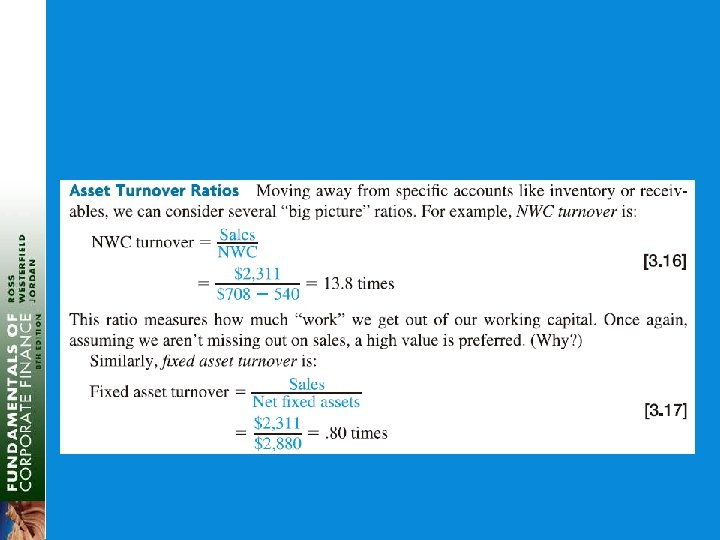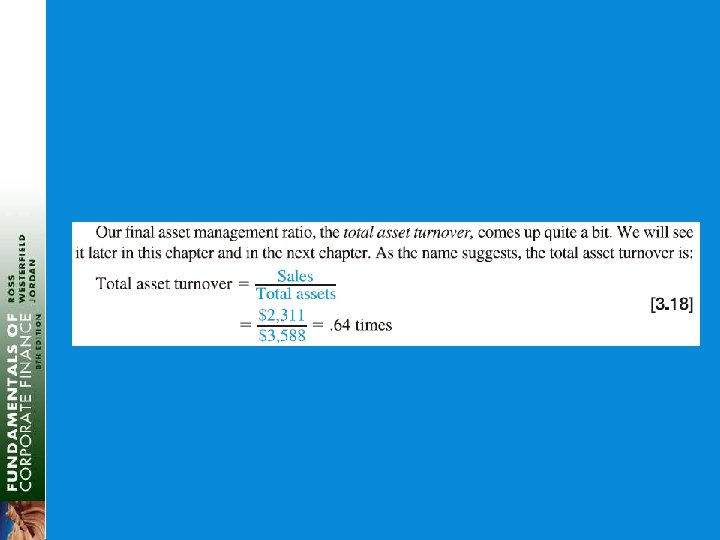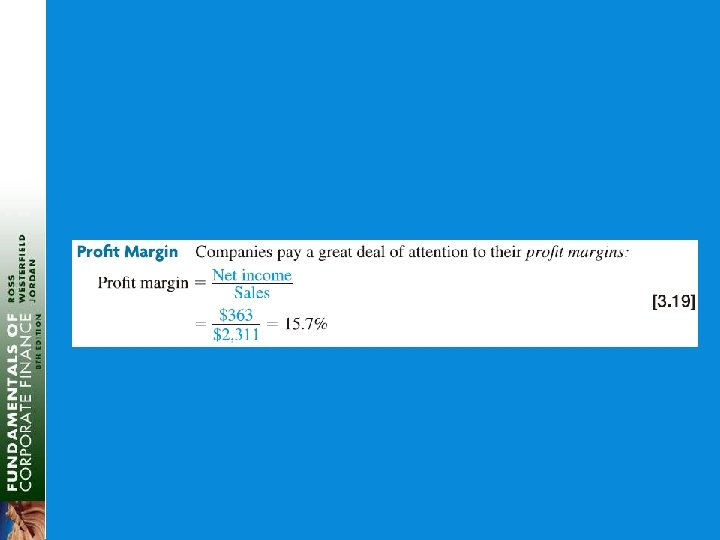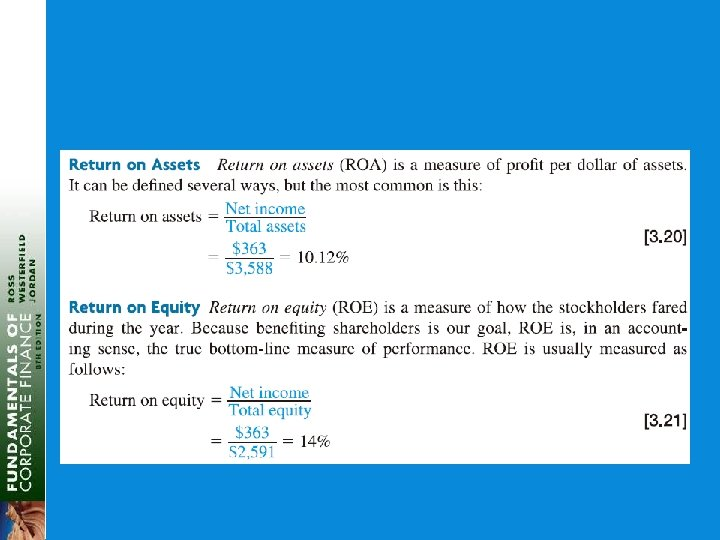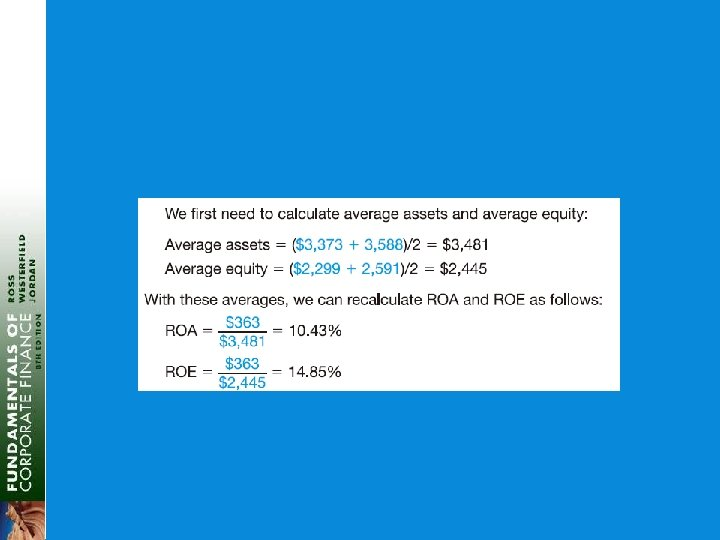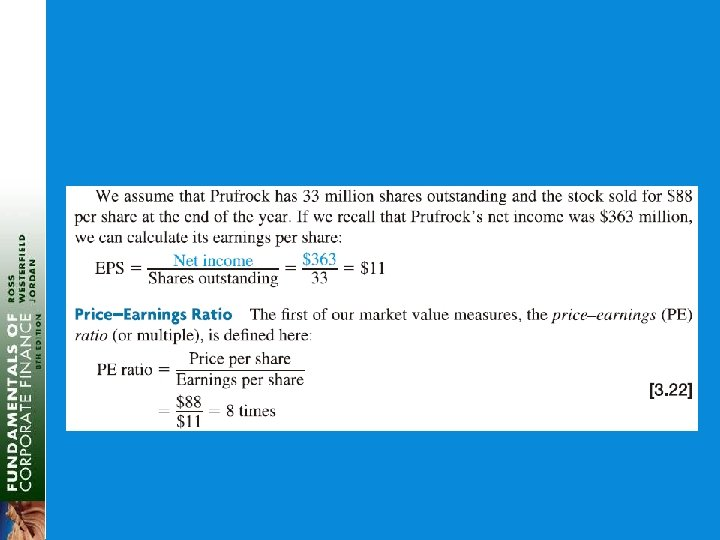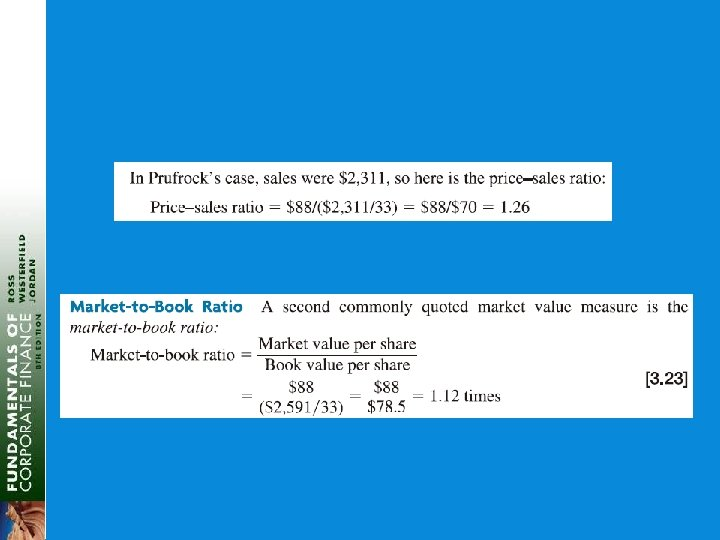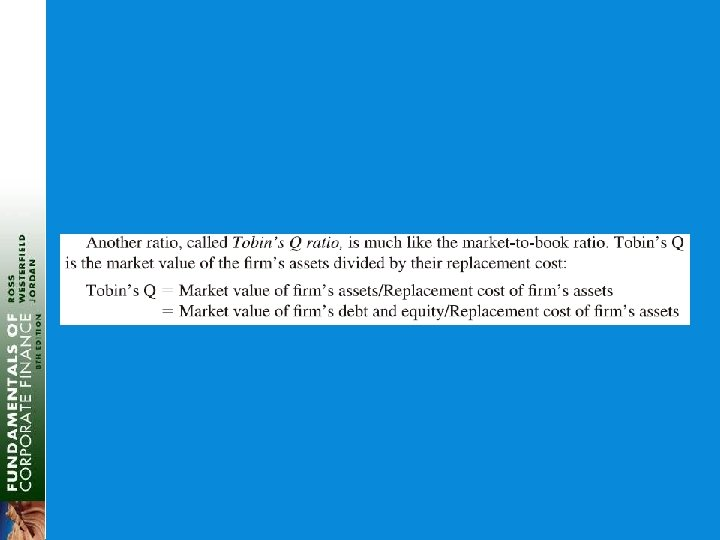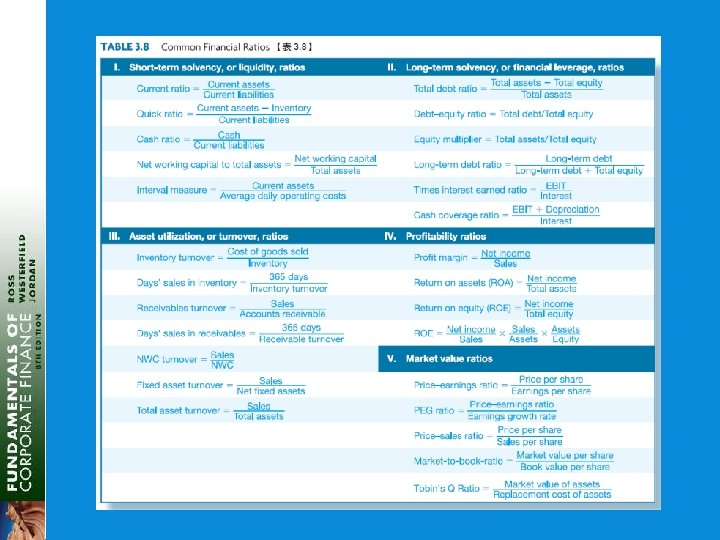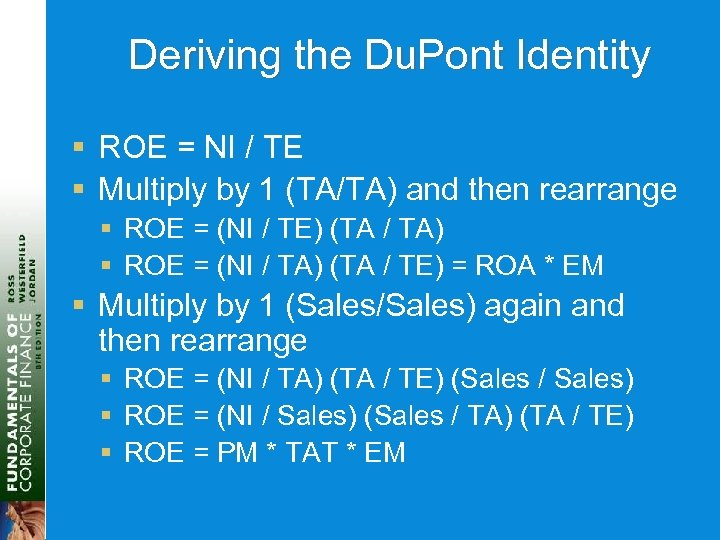Deriving the Du. Pont Identity § ROE = NI / TE § Multiply by 1 (TA/TA) and then rearrange § ROE = (NI / TE) (TA / TA) § ROE = (NI / TA) (TA / TE) = ROA * EM § Multiply by 1 (Sales/Sales) again and then rearrange § ROE = (NI / TA) (TA / TE) (Sales / Sales) § ROE = (NI / Sales) (Sales / TA) (TA / TE) § ROE = PM * TAT * EM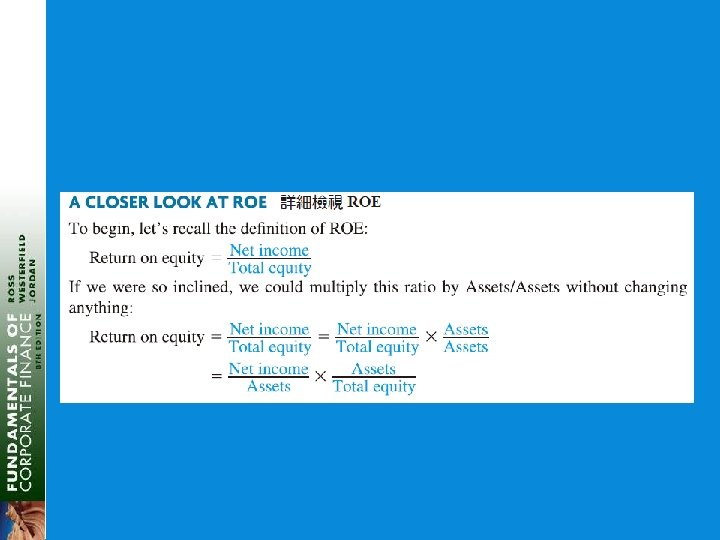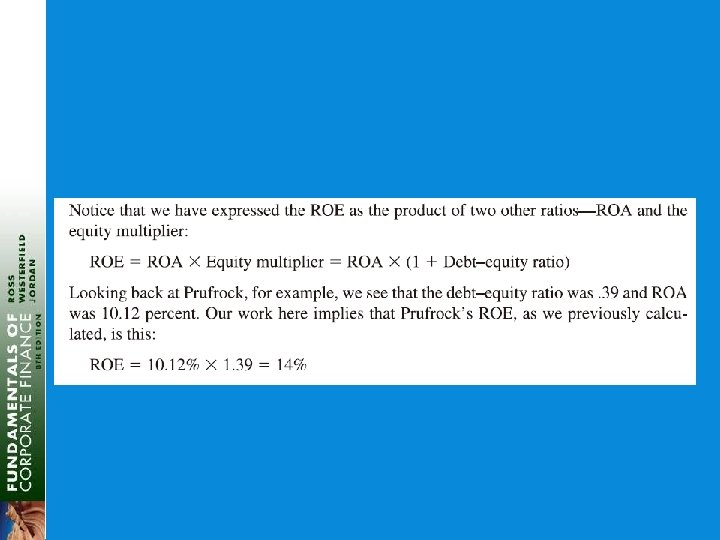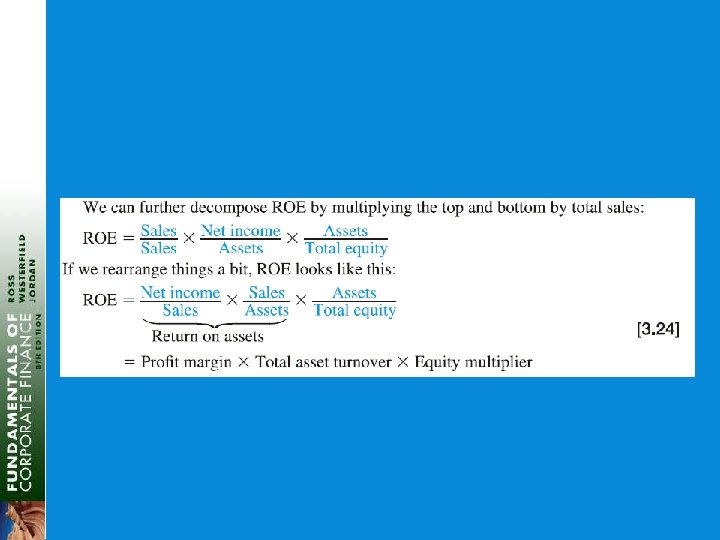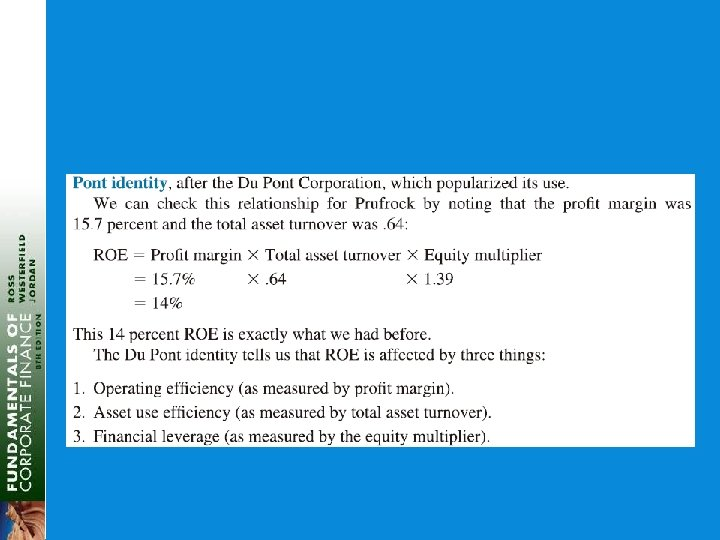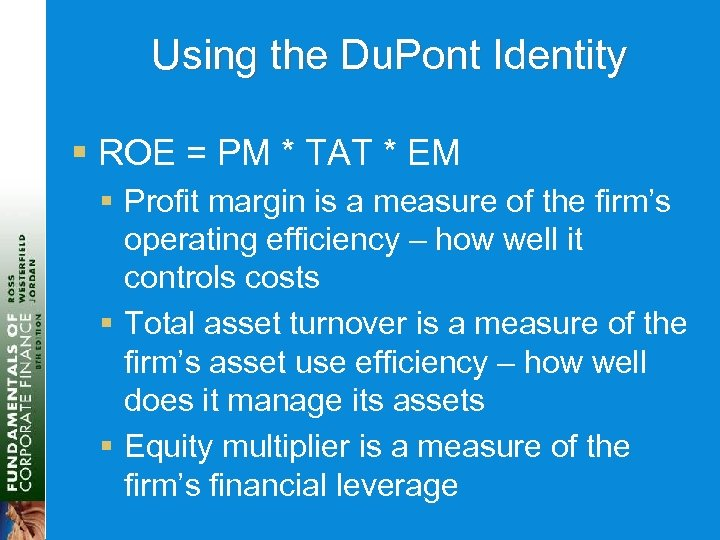Using the Du. Pont Identity § ROE = PM * TAT * EM § Profit margin is a measure of the firm’s operating efficiency – how well it controls costs § Total asset turnover is a measure of the firm’s asset use efficiency – how well does it manage its assets § Equity multiplier is a measure of the firm’s financial leverage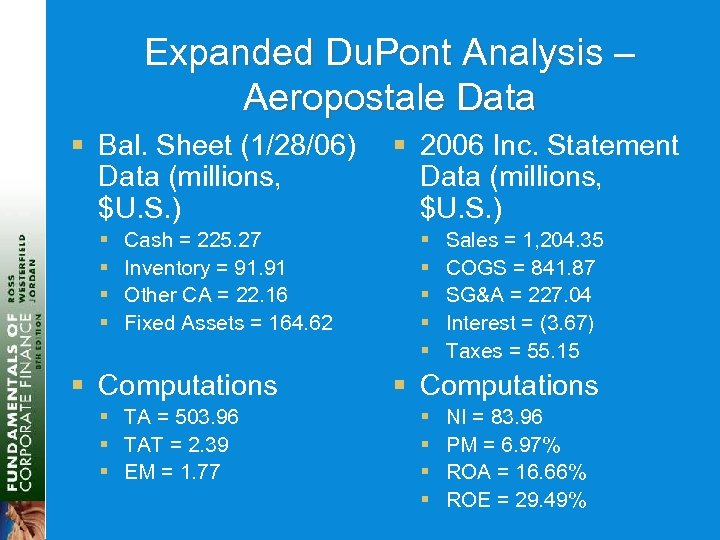Expanded Du. Pont Analysis – Aeropostale Data § Bal. Sheet (1/28/06) Data (millions, \$U. S. ) § § Cash = 225. 27 Inventory = 91. 91 Other CA = 22. 16 Fixed Assets = 164. 62 § Computations § TA = 503. 96 § TAT = 2. 39 § EM = 1. 77 § 2006 Inc. Statement Data (millions, \$U. S. ) § § § Sales = 1, 204. 35 COGS = 841. 87 SG&A = 227. 04 Interest = (3. 67) Taxes = 55. 15 § Computations § § NI = 83. 96 PM = 6. 97% ROA = 16. 66% ROE = 29. 49%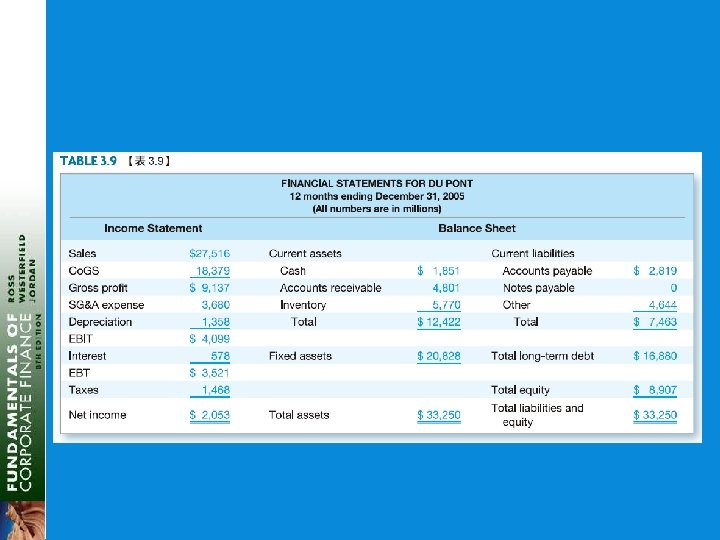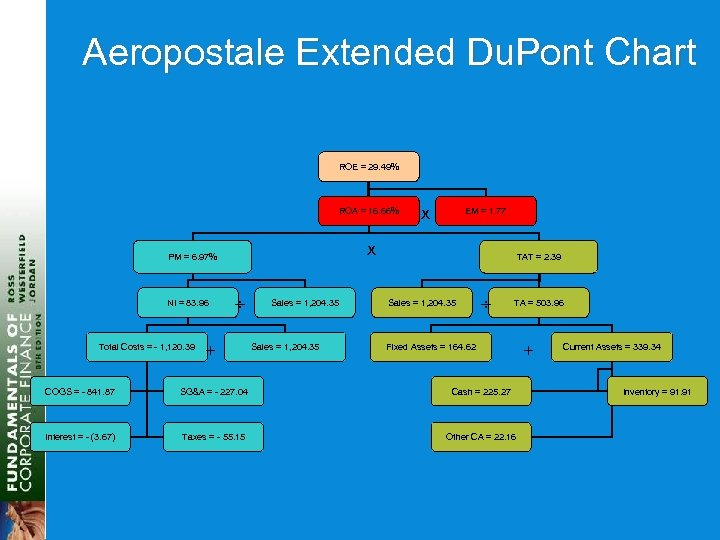Aeropostale Extended Du. Pont Chart ROE = 29. 49% ROA = 16. 66% Total Costs = - 1, 120. 39 EM = 1. 77 x PM = 6. 97% NI = 83. 96 x + Sales = 1, 204. 35 TAT = 2. 39 Sales = 1, 204. 35 TA = 503. 96 Fixed Assets = 164. 62 COGS = - 841. 87 SG&A = - 227. 04 Cash = 225. 27 Interest = - (3. 67) Taxes = - 55. 15 Other CA = 22. 16 + Current Assets = 339. 34 Inventory = 91. 91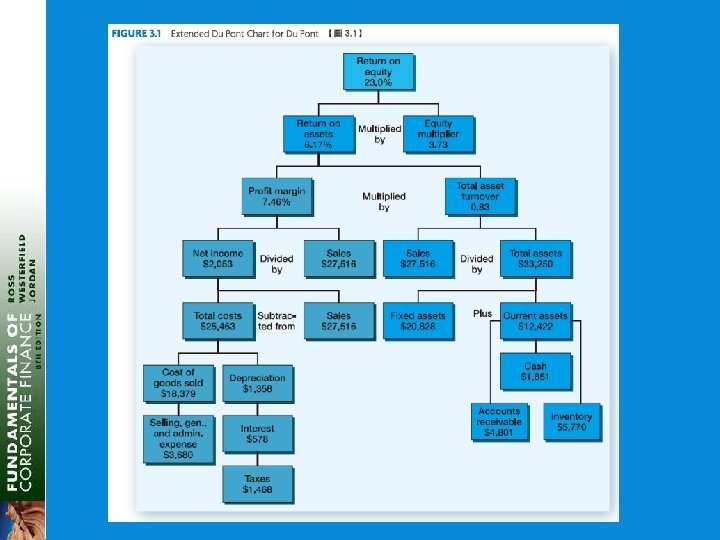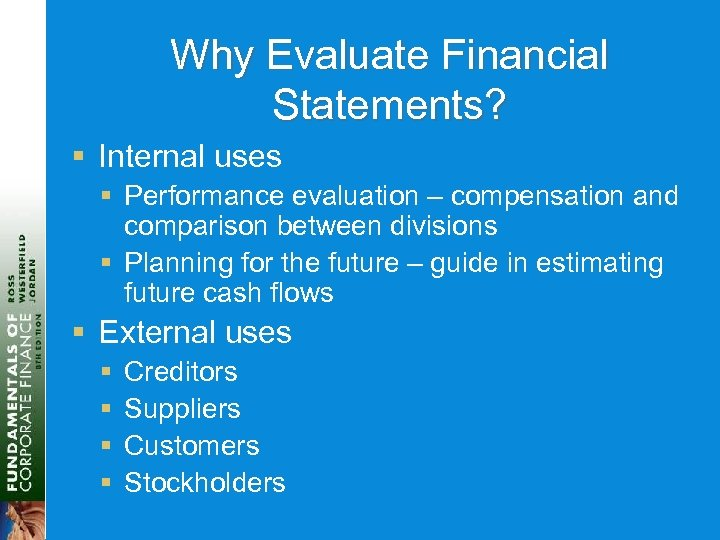Why Evaluate Financial Statements? § Internal uses § Performance evaluation – compensation and comparison between divisions § Planning for the future – guide in estimating future cash flows § External uses § § Creditors Suppliers Customers Stockholders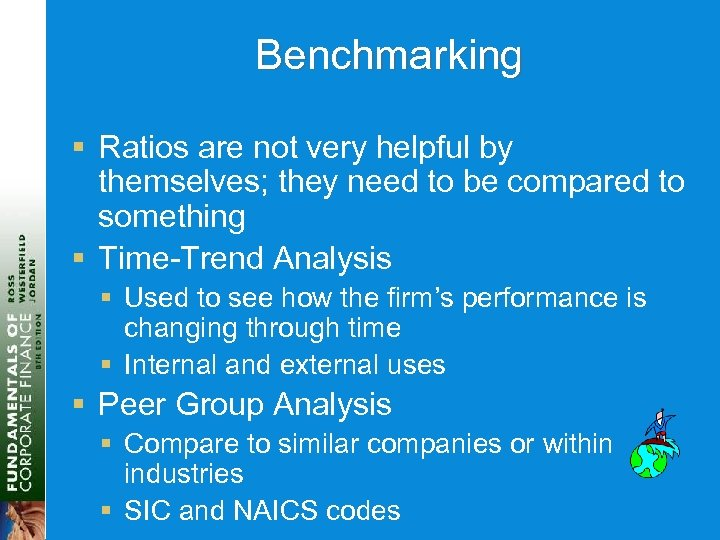Benchmarking § Ratios are not very helpful by themselves; they need to be compared to something § Time-Trend Analysis § Used to see how the firm’s performance is changing through time § Internal and external uses § Peer Group Analysis § Compare to similar companies or within industries § SIC and NAICS codes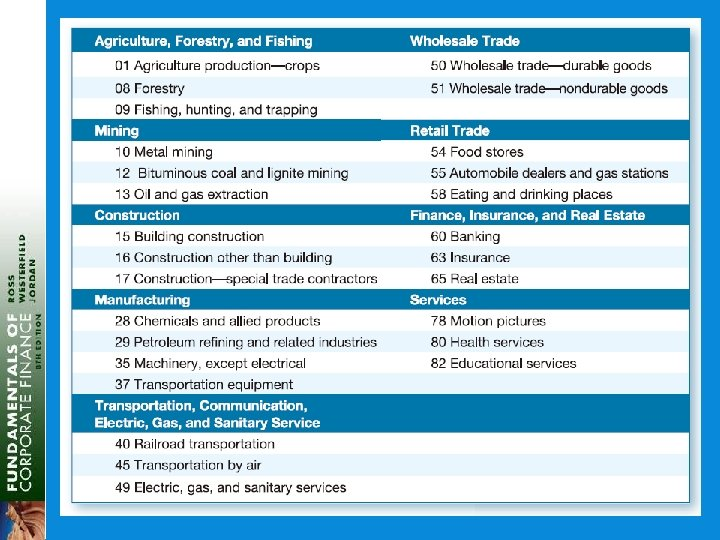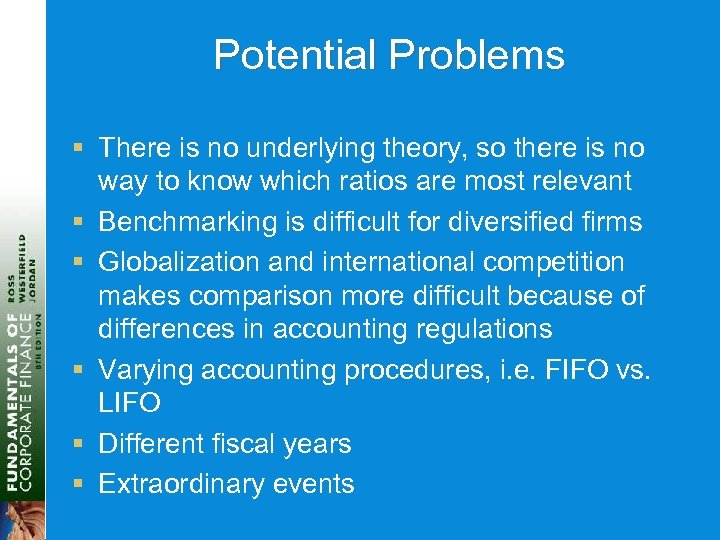Potential Problems § There is no underlying theory, so there is no way to know which ratios are most relevant § Benchmarking is difficult for diversified firms § Globalization and international competition makes comparison more difficult because of differences in accounting regulations § Varying accounting procedures, i. e. FIFO vs. LIFO § Different fiscal years § Extraordinary events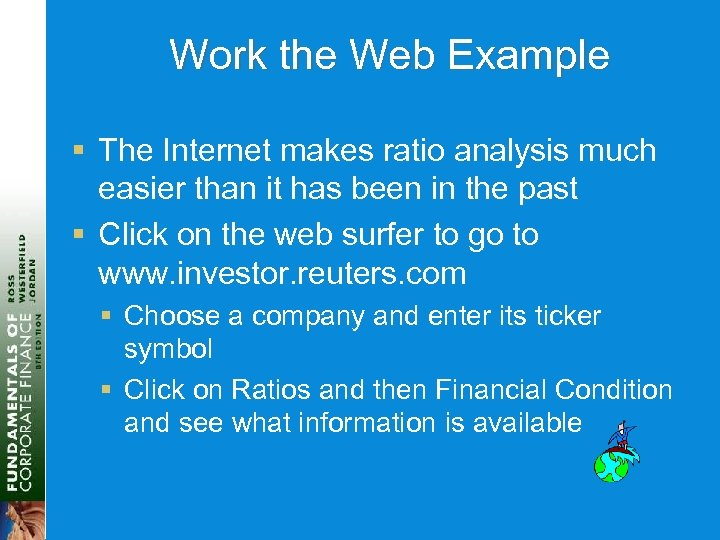Work the Web Example § The Internet makes ratio analysis much easier than it has been in the past § Click on the web surfer to go to www. investor. reuters. com § Choose a company and enter its ticker symbol § Click on Ratios and then Financial Condition and see what information is available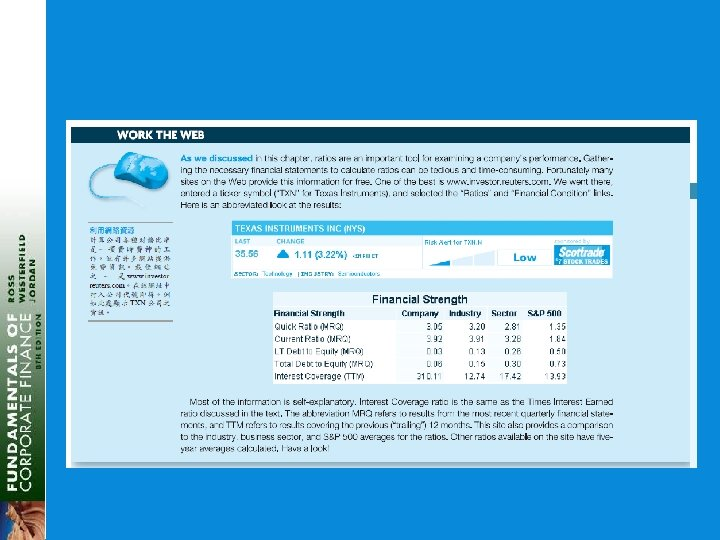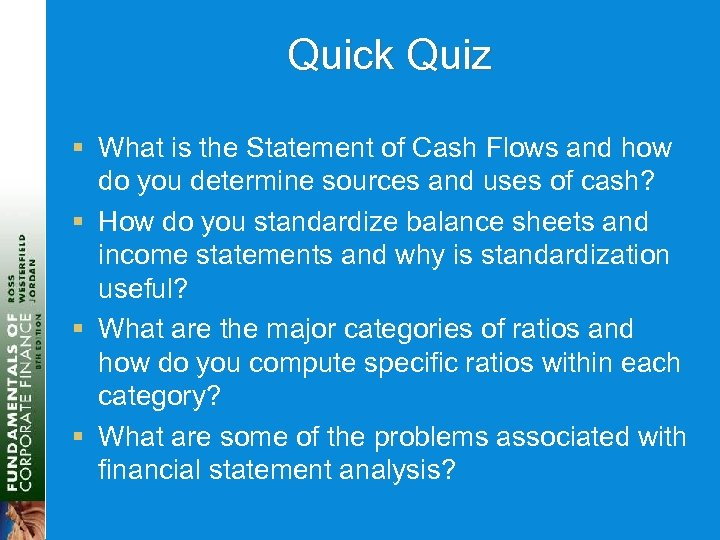Quick Quiz § What is the Statement of Cash Flows and how do you determine sources and uses of cash? § How do you standardize balance sheets and income statements and why is standardization useful? § What are the major categories of ratios and how do you compute specific ratios within each category? § What are some of the problems associated with financial statement analysis?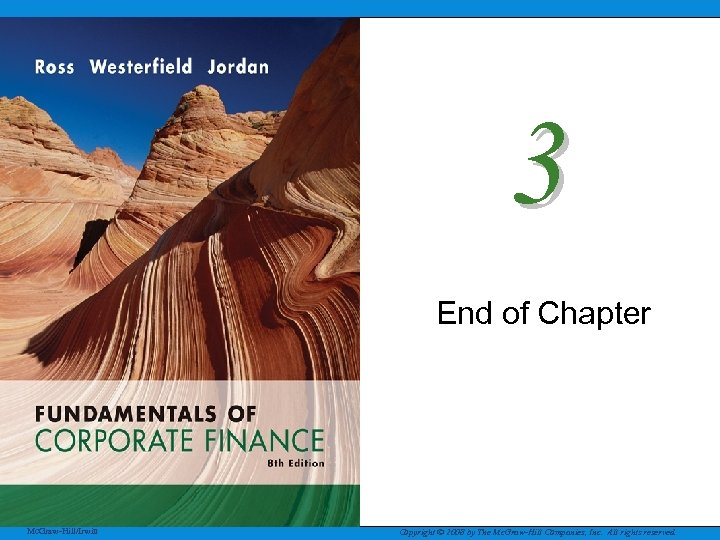3 End of Chapter Mc. Graw-Hill/Irwin Copyright © 2008 by The Mc. Graw-Hill Companies, Inc. All rights reserved.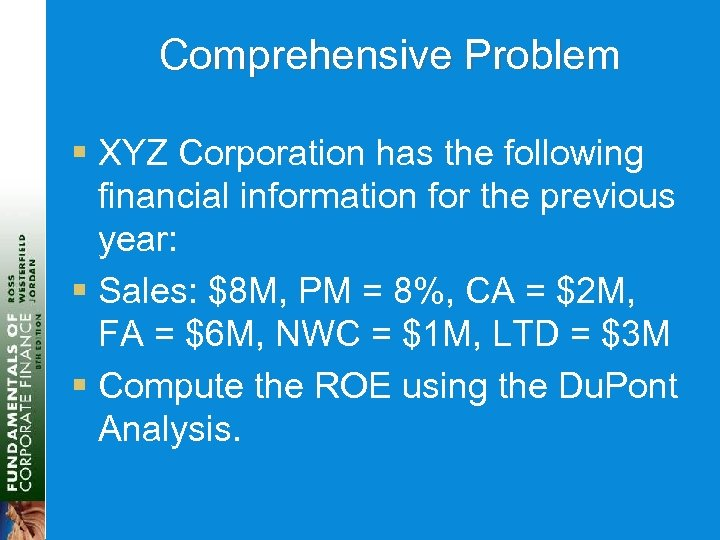Comprehensive Problem § XYZ Corporation has the following financial information for the previous year: § Sales: \$8 M, PM = 8%, CA = \$2 M, FA = \$6 M, NWC = \$1 M, LTD = \$3 M § Compute the ROE using the Du. Pont Analysis.